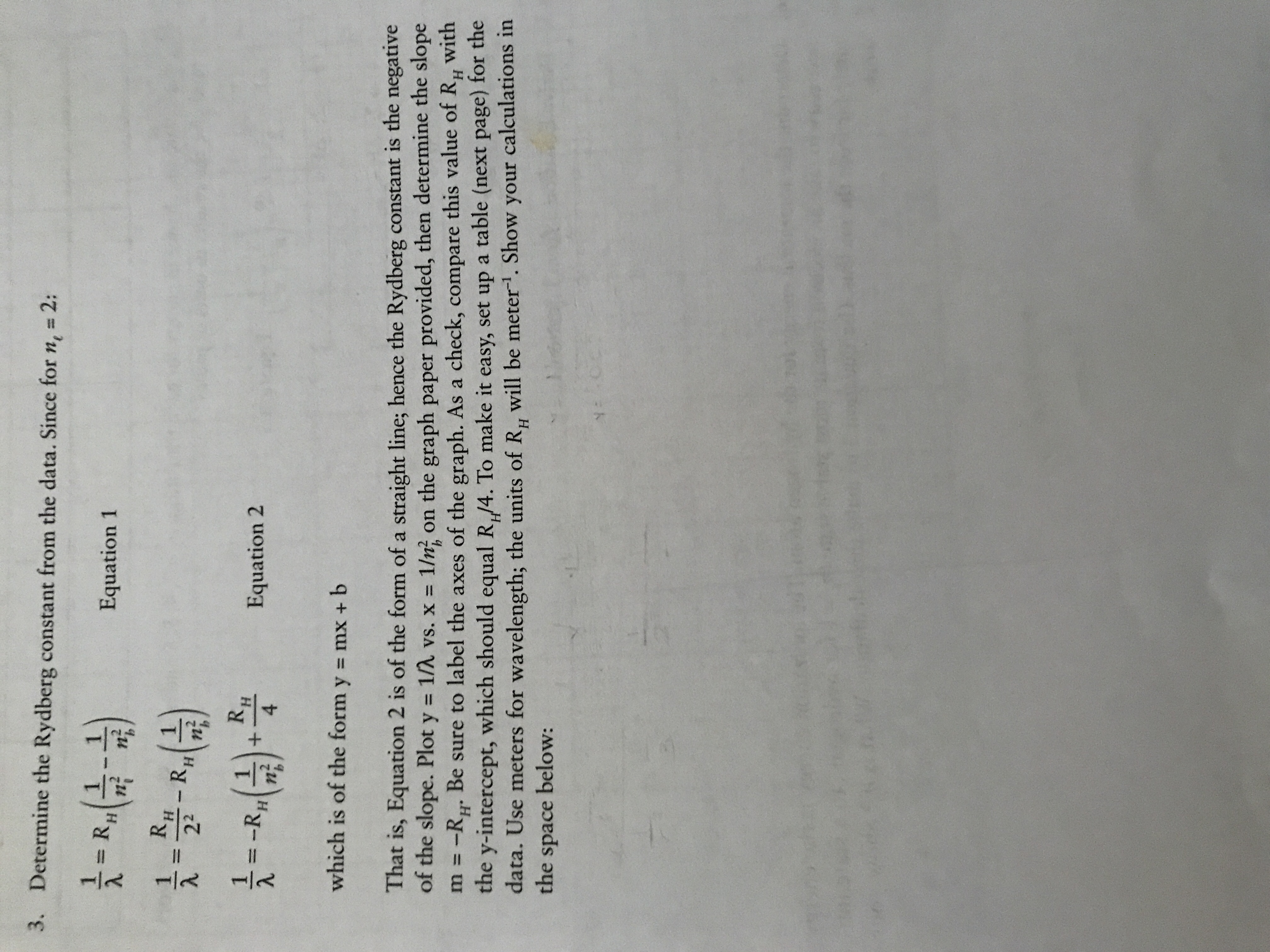# 3, Determine the Rydberg constant from the data. Since for n1 = 2: tad**) Equation 1 릇=-R-G)- Equation 2 4 which is of the form y - mx+ b That is, Equation 2 is of the form of a straight line, hence the Rydberg constant is the negative of the slope. Plot y 1/0 vs. x 1/ni on the graph paper provided, then determine the slope m =-R," Be sure to label the axes of the graph. As a check, compare this value of RH with the y-intercept, which should equal R,(4. To make it easy, set up a table (next page) for the data. Use meters for wavelength; the units of R, will be meter". Show your calculations in the space below:

Questionhelp_outlineImage Transcriptionclose3, Determine the Rydberg constant from the data. Since for n1 = 2: tad**) Equation 1 릇=-R-G)- Equation 2 4 which is of the form y - mx+ b That is, Equation 2 is of the form of a straight line, hence the Rydberg constant is the negative of the slope. Plot y 1/0 vs. x 1/ni on the graph paper provided, then determine the slope m =-R," Be sure to label the axes of the graph. As a check, compare this value of RH with the y-intercept, which should equal R,(4. To make it easy, set up a table (next page) for the data. Use meters for wavelength; the units of R, will be meter". Show your calculations in the space below: fullscreen

### Want to see this answer and more?

Experts are waiting 24/7 to provide step-by-step solutions in as fast as 30 minutes!*